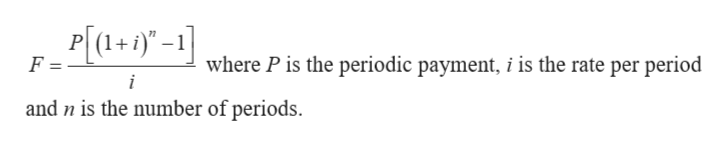# I am working on annuities and this one annuity is really difficult. the question is Kristy Joe deposits \$160 each month in an ordinary annuity to save for a new car. If the annuity pays a monthly interest rate of 0.66% how much will she be able to save in 5 years? Assume that the compounding is being done monthly.The answer I have come up with are 11,337.04 but the correct answer is apparently 11,731.81. How is this possible?

Question
30 views

I am working on annuities and this one annuity is really difficult.

the question is Kristy Joe deposits \$160 each month in an ordinary annuity to save for a new car. If the annuity pays a monthly interest rate of 0.66% how much will she be able to save in 5 years? Assume that the compounding is being done monthly.

The answer I have come up with are 11,337.04 but the correct answer is apparently 11,731.81. How is this possible?

check_circle

Step 1

Given information:

Periodic payment (P)= \$160

Rate of interest (i) = 0.66% = 0.0066

Compounded monthly (n) = (5)(12) = 60

Step 2

Future value of annuity formula:

...help_outlineImage TranscriptioncloseP[+1)-1 where P is the periodic payment, i is the rate per period F i and n is the number of periods. fullscreen

### Want to see the full answer?

See Solution

#### Want to see this answer and more?

Solutions are written by subject experts who are available 24/7. Questions are typically answered within 1 hour.*

See Solution
*Response times may vary by subject and question.
Tagged in

### Math